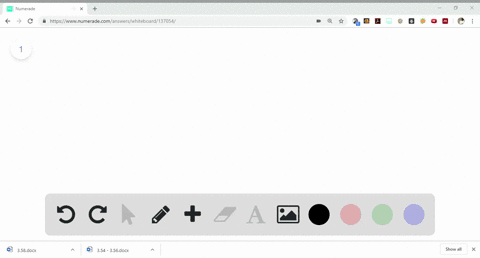Sign up for our free STEM online summer camps starting June 1st!View Summer Courses## Discussion

You must be signed in to discuss.

## Video Transcript

let's start. Firstly, we need to calculate the mass off CEO and IT Adam in carbon dioxide and water, respectively. So the moss off carbon is 0.66 to 7 grams by the mass off hydrogen is 0.561 ground. To determine the mass off oxygen, we just simply data mine. We just simply subtract the mass off fire carbon on hydrogen from the total mouth off the sample, which gives us the mask off oxygen as 0.33 grounds. Now we need to calculate the moles off each element. The formula to data mine. The number off moves off each element. Is weight divided by the molecular mass. Using this formula, we can data mine the number off moves off carbon, hydrogen and oxygen. No to data mine. This impressed bull number ratio by dividing each mole by 0.7 mole because so for carbon it is 0.0 552 divided by 0.207 which gives US 2.66 for hydrogen, it is 0.554 divided by 0.0 207 gives us 2.67 For excision, it is 0.207 divided by 0.207 Gives us what the subscript off carbon and hydrogen are not. Wool number saw him, so we multiplied them by three to get the whole number. So multiplied them by three. Which gives us a goblin as eight Hydro Jinnah's eight excision as three. Which gives us the final empirical formula off the molecule compound. I see it. It's speed 43